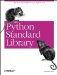# The array Module

The array module implements an efficient array storage type. Arrays are similar to lists, but all items must be of the same primitive type. The type is defined when the array is created.

Examples 4-1 through 4-5 are simple ones. Example 4-1 creates an array object and copies the internal buffer to a string through the tostring method.

Example 4-1. Using the array Module to Convert Lists of Integers to Strings

```File: array-example-1.py

import array

a = array.array("B", range(16)) # unsigned char
b = array.array("h", range(16)) # signed short

print a
print repr(a.tostring())

print b
print repr(b.tostring())

array('B', [0, 1, 2, 3, 4, 5, 6, 7, 8, 9, 10, 11, 12, 13, 14, 15])
'00010203040506071011121314151617'

array('h', [0, 1, 2, 3, 4, 5, 6, 7, 8, 9, 10, 11, 12, 13, 14, 15])
'00000100020003000400050006000700
10001100120013001400150016001700'```

The array objects can be treated as ordinary lists to some extent, as Example 4-2 shows. You cannot concatenate arrays if they have different type codes, though.

Example 4-2. Using Arrays as Ordinary Sequences

```File: array-example-2.py

import array

a = array.array("B", [1, 2, 3])

a.append(4)

a = a + a

a = a[2:-2]

print a
print repr(a.tostring())
for i in a:
print i,

array('B', [3, 4, 1, 2])
'03040102'
3 4 1 2```

This module also provides a very efficient way to turn raw binary data into a sequence of integers (or floating point values, for that matter), as Example 4-3 demonstrates.

Example 4-3. Using Arrays to Convert Strings to Lists of Integers

```File: array-example-3.py

import array

a = array.array("i", "fish license") # signed integer

print a
print repr(a.tostring())
print a.tolist()

array('i', [1752394086, 1667853344, 1702063717])
[1752394086, 1667853344, 1702063717]```

Finally, Example 4-4 shows how to use this module to determine the endianess of the current platform.

Example 4-4. Using the array Module to Determine Platform Endianess

```File: array-example-4.py

import array

def little_endian():
return ord(array.array("i",).tostring())

if little_endian():
print "little-endian platform (intel, alpha)"
else:
print "big-endian platform (motorola, sparc)"

big-endian platform (motorola, sparc)```

Python 2.0 and later provides a sys.byteorder attribute, which is set to either "little" or "big," as you can see in Example 4-5.

Example 4-5. Using the sys.byteorder Attribute to Determine Platform Endianess (Python 2.0)

```File: sys-byteorder-example-1.py

import sys

# 2.0 and later
if sys.byteorder == "little":
print "little-endian platform (intel, alpha)"
else:
print "big-endian platform (motorola, sparc)"

big-endian platform (motorola, sparc)```Python Standard Library (Nutshell Handbooks) with
ISBN: 0596000960
EAN: 2147483647
Year: 2000
Pages: 252
Authors: Fredrik Lundh